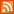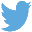Bucaro TecHelpHTTPS Encryption not required because no account numbers orpersonal information is ever requested or accepted by this siteCustom Search# Display a Value in Currency Format

To display a currency value with the proper number of digits to the right of the decimal point, first use the Math.pow function to calculate a multiplier, then use that multiplier to round the number to the required number of digits.

Convert that value to a string so you can determine the location of the decimal point, or if you need to add a decimal point, and if you need to add zeros to the decimal portion. Code to convert a value to currency format is shown below.

```<script>
function formatTo(value, precision)
{
// calculate multiplier required to get required number
// of digits to the right of the decimal point
var multiplier = Math.pow(10, precision);

// round value to required number of digits
var amount = Math.round(value * multiplier) / multiplier;

// convert to string
var strAmt = amount.toString();

// determine if you need to add zeros to decimal part
var decimalIndex = strAmt.indexOf(".");
if(precision > 0 && decimalIndex < 0)
{
decimalIndex = strAmt.length;
strAmt += '.';
}

// add any required zeros to decimal part
while(decimalIndex + precision + 1 > strAmt.length)
{
strAmt += '0';
}
return strAmt;
}

document.write(formatTo(4.003, 2));
</script>
```

Call the formatTo function, passing the value to format and the precision (number of digits to the right of the decimal point.

 .menubtn { margin-top:10px; margin-left:50px; width:220px; opacity:1; border-style:solid; border-color:#009000; background-color:#66ff66; transition-property: opacity; transition-duration: 1s; transition-timing-function: ease-out; } .menubtn:hover { opacity:1; background-color:#e5ff23; } Menu - More Java Script ProgrammingRSS FeedFollow @Stephen Bucaro[Site User Agreement] [Privacy Policy] [Site map] [Search This Site] [Contact Form]
Copyright©2001-2020 Bucaro TecHelp 13771 N Fountain Hills Blvd Suite 114-248 Fountain Hills, AZ 85268